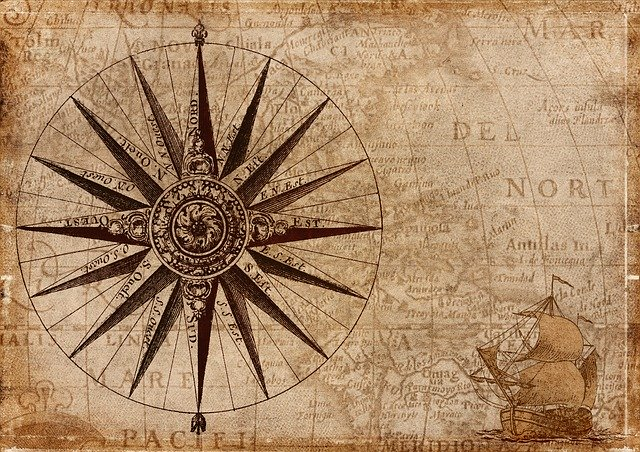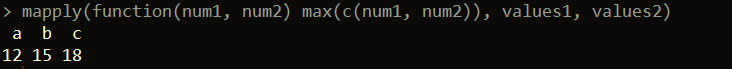Want to share your content on R-bloggers? click here if you have a blog, or here if you don't.An older post on this blog talked about several alternative base apply functions. This post will talk about how to apply a function across multiple vectors or lists with Map and mapply in R. These functions are generalizations of sapply and lapply, which allow you to more easily loop over multiple vectors or lists simultaneously.

## Map

Suppose we have two lists of vectors and we want to divide the nth vector in one list by the nth vector in the second list. Map makes this straightforward to accomplish, while keeping the code clean to read. Map returns a list by default, similar to lapply.

Below, we create two sample lists of vectors.

```values1 <- list(a = c(1, 2, 3), b = c(4, 5, 6), c = c(7, 8, 9))

values2 <- list(a = c(10, 11, 12), b = c(13, 14, 15), c = c(16, 17, 18))

```

Now, let’s do the operation we described above using Map. Here, we’ll input the function as the first parameter. In this case, the function takes two numeric values as input and divides the first value by the second. The remaining inputs to Map are the names of the lists we are looping over.

```Map(function(num1, num2) num1 / num2, values1, values2)

```

num1 refers to each individual element in the iteration over values1, while num2 refers to each individual element in the iteration over values2. Each element in each list is a vector.Below is another example. Here, we loop over our two lists of vectors, and get the pairwise union of the vectors across the lists.

```Map(function(num1, num2) union(num1, num2), values1, values2)

```

## mapply

mapply, similar to sapply, tries to return a vector result when possible. Like Map, one difference between mapply and sapply or lapply is that the function to be applied is input as the first parameter.

Let’s suppose we again have our two lists of vectors, but this time we want to get the maximum value across two pairwise vectors for each pair of vectors in the lists.

```mapply(function(num1, num2) max(c(num1, num2)), values1, values2)

```Here, mapply loops over each of the lists simultaneously. For the nth vector in each list, mapply combines the two vectors and finds the maximum value.

Map is actually a wrapper around mapply, with the parameter SIMPLIFY set to FALSE. Setting this parameter to TRUE (which is default) means (as mentioned above) mapply will try to simplify the result to a vector if possible. Each of these functions can also be useful in iterating over lists of data frames.